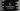# C++ program to find armstrong numbers in a range# Introduction :

Armstrong numbers are numbers with a sum of each digit, each raised to the power of the number of digits is equal to the number itself. For example, 153 is an Armstrong number. The total digits of 153 are 3. Sum of all digits each raised to 3 is :

``````1^3 + 5^3 + 3^3
= 1 + 125 + 27
= 153``````

i.e. it is equal to the number itself. So, it is an Armstrong number.

In this post, we will learn how to find all Armstrong numbers in a range using C++, i.e. the user will provide the start and end position and the program will find out all numbers within these positions.

## C++ example program :

### Approach 1: Without using any library header :

``````#include <iostream>
using namespace std;

int findLength(int number)
{
int length = 0;

while (number != 0)
{
length++;
number /= 10;
}
return length;
}

int findPower(int number, int power)
{
int result = 1;
while (power != 0)
{
result *= number;
power--;
}
return result;
}

int main()
{
int start;
int end;
int length;
int number;
int sum;

cout << "Enter start position : " << endl; cin >> start;

cout << "Enter the end position : " << endl; cin >> end;

for (int i = start; i < end; i++)
{
number = i;
sum = 0;
length = findLength(number);

while (number != 0)
{
sum += findPower(number % 10, length);
number /= 10;
}

if (i == sum)
{
cout << i << " ";
}
}

return 0;
}``````

### Approach 2: Using cmath :

``````#include <iostream>
#include <cmath>
using namespace std;

int main()
{
int start;
int end;
int length;
int number;
int sum;

cout << "Enter start position : " << endl; cin >> start;

cout << "Enter the end position : " << endl; cin >> end;

for (int i = start; i < end; i++)
{
number = i;
sum = 0;
length = to_string(number).length();

while (number != 0)
{
sum += pow(number % 10, length);
number /= 10;
}

if (i == sum)
{
cout << i << " ";
}
}

return 0;
}``````

This is similar to the above example. The only differences are finding the length and finding the power. We are converting the integer to string to find the length. For power, we are using the pow method.

It will print the same output as the above example.